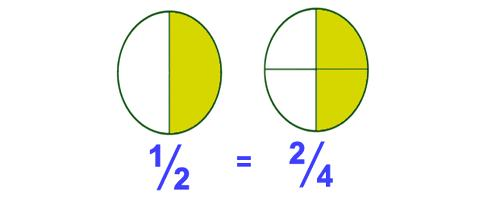Comparing Fractions with the Same Denominator

A Fraction consists of two numbers separated by a line. Comparing fractions is not as straight forward as comparing common numbers. In a fraction the numbers may appear to be larger but in fraction form their real value could be smaller. For example, 13/20 may appear bigger than 3/4 but in actual fact it is smaller.

The top number (or numerator) tells how many fractional pieces there are. In the fraction 3/8, we have three pieces.

The denominator of a fraction tells how many pieces an object was divided into. The fraction 3/8 tells us that the whole object was divided into 8 pieces.

If the denominators of two fractions are the same, the fraction with the largest numerator is the larger fraction.

For example 5/8 is larger than 3/8 because all of the pieces are the same and five pieces are more than three pieces.

A fraction always refers to part of a whole thing. So, a fraction like 3/4 means three quarters of a thing. It could mean three quarters of a glass of water or three quarters of the population or three quarters of a footy match. When comparing fractions we must first make sure that they parts of the same number the whole thing was divided into. This means to compare fractions, they must first have the same denominator.How to Compare Fractions

To compare fractions with unlike denominators convert them to equivalent fractions with the same denominator.

• If you have mixed numbers convert them to improper fractions
• Find the lowest common denominator (LCD) for the fractions
• Convert each fraction into its equivalent with the LCD in the denominator
• Compare fractions: If denominators are the same you can compare the numerators. The fraction with the bigger numerator is the larger fraction.

Example:

Compare 5/6 and 3/8.

Find the LCD: The multiples of 6 are 6, 12, 18, 24, 30, etc. The multiples of 8 are 8, 16, 24, 32, etc. The lowest common multiple is 24 so we use that as the lowest common denominator.

Convert each fraction to its equivalent fraction using the LCD.

For 5/6, multiply numerator and denominator by 4 to have LCD = 24 in the denominator.

Information Source: